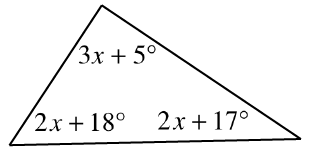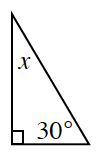### Home > INT1 > Chapter 10 > Lesson 10.1.4 > Problem10-69

10-69.

For each diagram below, solve for $x$. Explain what angle relationship(s) you used.

1.Review the Math Notes box in section 7.1.2

Since the angles of a triangle add up to $180º$, set up an equation using all three angles.

1.$x = 60º$